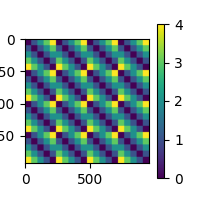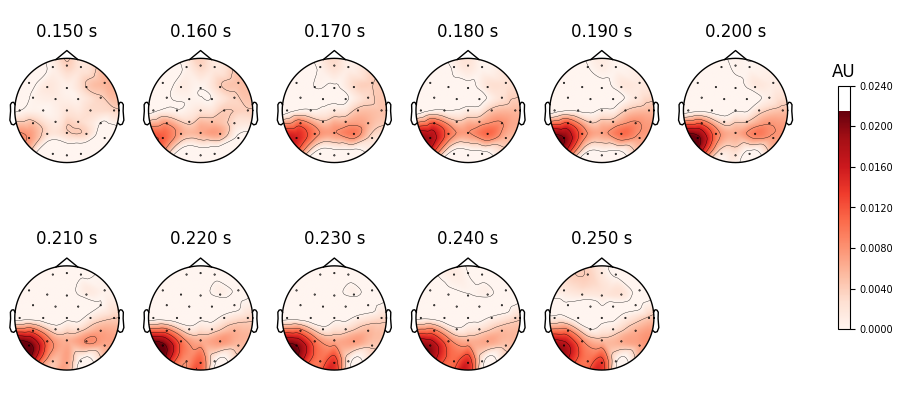# Sensor-level RSA using a searchlight¶

This example demonstrates how to perform representational similarity analysis (RSA) on EEG data, using a searchlight approach.

In the searchlight approach, representational similarity is computed between the model and searchlight “patches”. A patch is defined by a seed point (e.g. sensor Pz) and everything within the given radius (e.g. all sensors within 4 cm. of Pz). Patches are created for all possible seed points (e.g. all sensors), so you can think of it as a “searchlight” that moves from seed point to seed point and everything that is in the spotlight is used in the computation.

The radius of a searchlight can be defined in space, in time, or both. In this example, our searchlight will have a spatial radius of 4.5 cm. and a temporal radius of 50 ms.

The dataset will be the kiloword dataset : approximately 1,000 words were presented to 75 participants in a go/no-go lexical decision task while event-related potentials (ERPs) were recorded.

```# sphinx_gallery_thumbnail_number=2

# Import required packages
import mne
import mne_rsa
```

MNE-Python contains a build-in data loader for the kiloword dataset. We use it here to read it as 960 epochs. Each epoch represents the brain response to a single word, averaged across all the participants. For this example, we speed up the computation, at a cost of temporal precision, by downsampling the data from the original 250 Hz. to 100 Hz.

```data_path = mne.datasets.kiloword.data_path(verbose=True)
epochs = epochs.resample(100)
```
```Reading C:\Users\wmvan\mne_data\MNE-kiloword-data\kword_metadata-epo.fif ...
Found the data of interest:
t =    -100.00 ...     920.00 ms
0 CTF compensation matrices available
960 matching events found
No baseline correction applied
0 projection items activated
```

The kiloword datas was erroneously stored with sensor locations given in centimeters instead of meters. We will fix it now. For your own data, the sensor locations are likely properly stored in meters, so you can skip this step.

```for ch in epochs.info["chs"]:
ch["loc"] /= 100
```

The `epochs` object contains a `.metadata` field that contains information about the 960 words that were used in the experiment. Let’s have a look at the metadata for 10 random words:

```epochs.metadata.sample(10)
```
WORD Concreteness WordFrequency OrthographicDistance NumberOfLetters BigramFrequency ConsonantVowelProportion VisualComplexity
597 female 4.40 3.019116 2.35 6.0 530.666667 0.500000 69.709111
366 infant 5.60 2.571709 2.10 6.0 616.000000 0.666667 56.831681
41 dune 5.30 1.397940 1.25 4.0 349.000000 0.500000 72.852817
687 exchange 3.95 2.888741 2.75 8.0 495.000000 0.625000 73.889893
63 rifle 6.15 2.457882 1.70 5.0 302.000000 0.600000 50.065915
889 exhibit 4.30 1.447158 2.80 7.0 265.285714 0.571429 61.354880
353 author 5.00 2.718502 2.40 6.0 399.166667 0.500000 61.993270
228 disarray 3.25 1.662758 3.45 8.0 617.875000 0.500000 62.390205
124 saddle 6.00 2.173186 1.70 6.0 519.666667 0.666667 72.100254
699 momentum 3.65 2.252853 3.55 8.0 513.000000 0.625000 75.949282

Let’s pick something obvious for this example and build a dissimilarity matrix (RDM) based on the number of letters in each word.

```rdm_vis = mne_rsa.compute_rdm(epochs.metadata[["NumberOfLetters"]], metric="euclidean")
mne_rsa.plot_rdms(rdm_vis)
``````<Figure size 200x200 with 2 Axes>
```

The above RDM will serve as our “model” RDM. In this example RSA analysis, we are going to compare the model RDM against RDMs created from the EEG data. The EEG RDMs will be created using a “searchlight” pattern. We are using squared Euclidean distance for our RDM metric, since we only have a few data points in each searchlight patch. Feel free to play around with other metrics.

```rsa_result = mne_rsa.rsa_epochs(
epochs,  # The EEG data
rdm_vis,  # The model RDM
epochs_rdm_metric="sqeuclidean",  # Metric to compute the EEG RDMs
rsa_metric="kendall-tau-a",  # Metric to compare model and EEG RDMs
tmin=0.15,
tmax=0.25,  # To save time, only analyze this time interval
n_jobs=1,  # Only use one CPU core. Increase this for more speed.
n_folds=None,  # Don't use any cross-validation
verbose=False,
)  # Set to True to display a progress bar
```
```Performing RSA between Epochs and 1 model RDM(s)
Using 29 sensors
Time interval: 0.15-0.25 seconds
Automatic dermination of folds: 1 (no cross-validation)
Creating spatio-temporal searchlight patches
```

The result is packed inside an MNE-Python `mne.Evoked` object. This object defines many plotting functions, for example `mne.Evoked.plot_topomap()` to look at the spatial distribution of the RSA values. By default, the signal is assumed to represent micro-Volts, so we need to explicitly inform the plotting function we are plotting RSA values and tweak the range of the colormap.

```rsa_result.plot_topomap(
rsa_result.times,
units=dict(eeg="kendall-tau-a"),
scalings=dict(eeg=1),
cbar_fmt="%.4f",
vlim=(0, None),
nrows=2,
sphere=1,
)
``````<MNEFigure size 1050x415 with 12 Axes>
```

Unsurprisingly, we get the highest correspondance between number of letters and EEG signal in areas in the visual word form area.

Total running time of the script: (0 minutes 24.291 seconds)

Gallery generated by Sphinx-Gallery